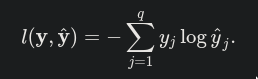# Softmax Regression Implementation from Scratch

The cross entropy loss is given by the below formula according to thisBut in the code below is the same thing happening?

``````def cross_entropy(y_hat, y):
return - np.log(y_hat[range(len(y_hat)), y])
``````

Am I missing something?

@gpk2000
You can test by your own next time…
Just try to input some numbers to see the outputs…

And use the search button as possible.http://preview.d2l.ai/d2l-en/master/chapter_appendix-mathematics-for-deep-learning/information-theory.html?highlight=cross%20entropy%20loss

`.mean()` is for n*1 matrix?
@goldpiggy

What this snippet of code is doing is exactly the same as the formula above. This code uses the index of the true y to fetch the predicted value y_hat and then taking the log to those predicted values for all examples in a minibatch

I am also a bit confused about that. I get that we select the y_hat for each y, in train_epoch_ch3 we sum over the cross-entropy loss, but where do we multiply each y with it’s corresponding y_hat as per the equation?

Hi @katduecker, great question! I guess you were referring to the “loss” function. Here we usedcross entropy loss rather than simply multiplying `y` and `y_hat` in the function `cross_entropy`.

Hi,
I think there is a typo in the sentence : “Before looking at the code, let us recall how this looks expressed as an equation:”
It should be : “Before looking at the code, let us recall how this looks when expressed as an equation:”
Thank you.
Nisar

I might be able to explain the derivation of the `cross_entropy` function a little bit.
Indeed, 𝓁(y,)=-Σyj log(j).
However, we see that y is in reality (0, … 0, 1, 0, …, 0) so we have:
𝓁(y,)=-Σyj log(j)=-yi log(i), where yi=1,
as all other terms are just zero.
Since yi=1, we can further simplify this into:
𝓁(y,)=-yi log(i)=-log(i), where yi=1.

``````def cross_entropy(y_hat, y):
return - np.log(y_hat[range(len(y_hat)), y])
``````

In the code, `y` is (i(1), i(2), …, i(n))
and `y_hat[range(len(y_hat)), y]` is just ((1)i(1), (2)i(2), …, (n)i(n))
with `-np.log` getting the logarithm of them all at once.

1 Like

softmax的一个稍微好一点的实现

``````import torch
def crossEntropyNaive(x):
expX = torch.exp(x)
return expX / expX.sum(axis = 1, keepdim=True)

def crossEntropySlightlySmart(x):
row_max,_ = torch.max(X, dim = 1, keepdim=True)
x = x - row_max
expX = torch.exp(x)
return expX / expX.sum(axis = 1, keepdim=True)

X = torch.tensor([[1.0,1.0],[1.0,10.0],[1.0,100.0]])
print(crossEntropyNaive(X))
print(crossEntropySlightlySmart(X))
``````